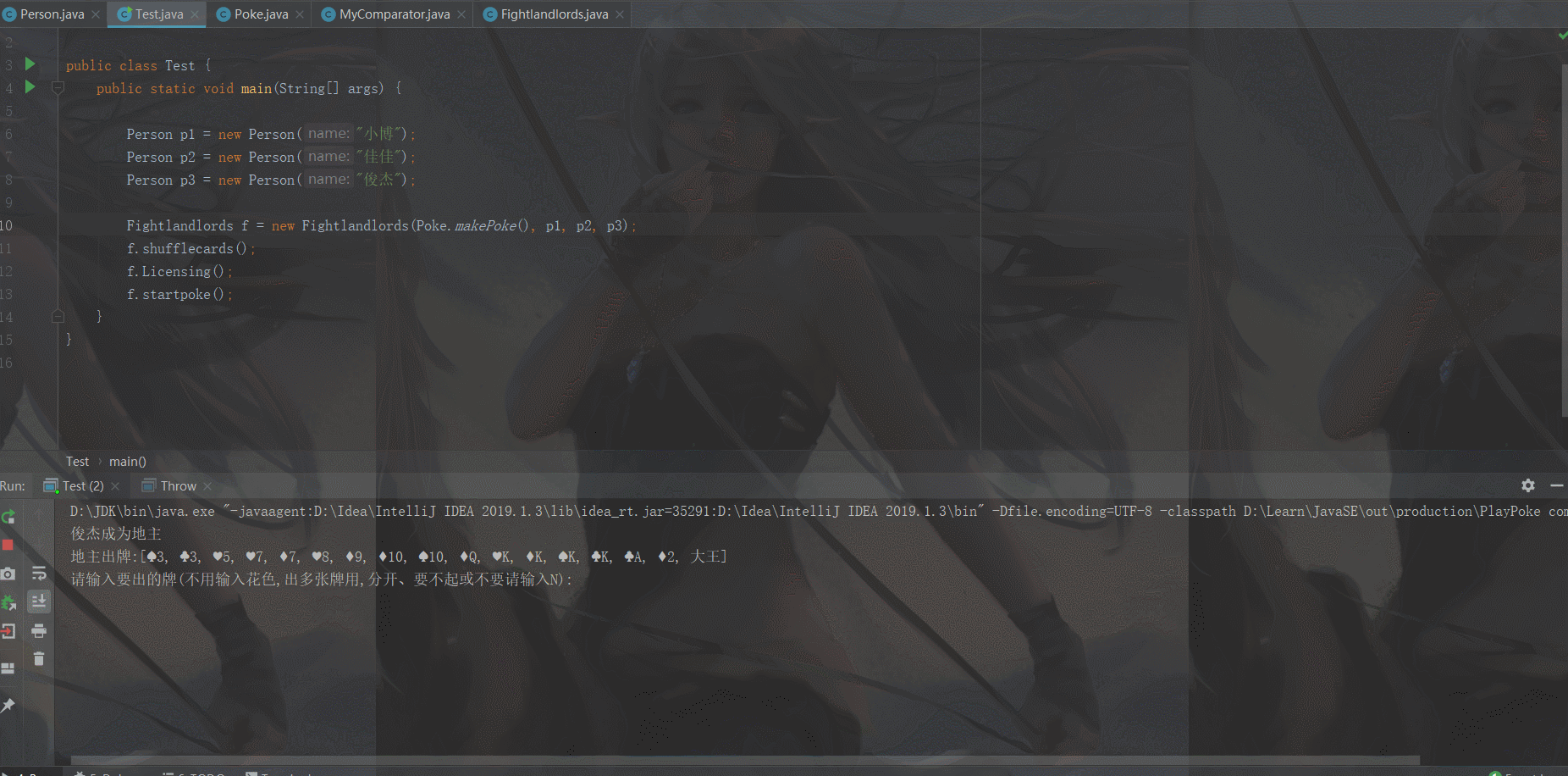# java實現輕輕松松控制臺鬥地主的示例代碼

## 1.先理清邏輯

• 需要創建一個牌類（Poke），它有牌號（pokecard）、花色（pokecolor）這兩個基本屬性，因為這兩個屬性是固定不改變的，所以在定義的時候我們可以把它們用final修飾符修飾。其次它還需要一個生成一副牌的方法makepoke()，以便於之後調用。隨後我們還需要有一個排序的方法，來進行一個從大到小的顯示，也方便在發牌之後重新進行排序。這裡就還有一個自定義的比較器接口MyComparator。
• 需要創建一個人物類（Person），它有一副牌（Poke）、人名(name)、是否是地主（islandlord）這三個基本屬性一個出牌的方法（sendpoke）
• 需要創建一個鬥地主的工具類（Fightlandlords），它有牌（Poke）、人（person）兩個基本屬性。一個選地主的方法（changelandlord）、一個洗牌的方法(shufflecards)、一個發牌的方法（Licensing）、一個打牌的方法（startpoke）。

## 按照思路一步步實現

```package com.dun.palypoke;
import java.util.ArrayList;
import java.util.Collections;

/*

*/
public class Poke{
private static final String[] pokecard = {“3”, “4”, “5”, “6”, “7”, “8”, “9”, “10”, “J”, “Q”, “K”, “A”, “2”, “小王”, “大王”}; //牌號
private static final String[] pokecolor = {“♠”, “♥”, “♣”, “♦”}; // 牌的花色

public String[] getPokecard() {
return pokecard;
}

public String[] getPokecolor() {
return pokecolor;
}

private Poke() {

}
/*
生成牌的方法， 返回一副牌
*/
public static ArrayList makePoke() {
ArrayList poke = new ArrayList<>();
for (int i = 0; i < pokecard.length - 2; i++) {
for (int j = 0; j < pokecolor.length; j++) {
}
}
/*
手動添加大小王
*/
return poke;
}
}``````package com.dun.palypoke;

import java.util.ArrayList;
import java.util.Iterator;
import java.util.Scanner;

/*

1.有一副牌(集合/數組)、名字、是否是地主三個屬性；
2.有出牌的方法，出牌前先在控制臺打印出所有的牌，再從控制臺接受出的牌，並出牌。
3.有理牌的方法，整理後牌按從小到大方式排序。

*/
public class Person {
private ArrayList poke = new ArrayList();         //一副牌
private String name;                             //名稱
private boolean islandlord;                     //是否是地主

public  Scanner sc = new Scanner(System.in);

public ArrayList getPoke() {
return poke;
}

public Person() {

}
public  Person(String name){
this.name = name;
}

public void setPoke(ArrayList poke) {
this.poke = poke;
}

public String getName() {
return name;
}

public void setName(String name) {
this.name = name;
}

public boolean isIslandlord() {
return islandlord;
}

public void setIslandlord(boolean islandlord) {
this.islandlord = islandlord;
}

/*
出牌     局部變量list的作用是當用戶出多張牌時，通過n++來表示用戶出的牌和他手上的牌是否全部存在，如果存在，就全部刪除。
*/
public ArrayList sendpoke(ArrayList poke){
System.out.println(poke);
ArrayList list = new ArrayList<>();         //臨時變量
for (int i = 0; i < poke.size(); i++) {
}
System.out.println("請輸入要出的牌(不用輸入花色,出多張牌用,分開、要不起或不要請輸入N):");
String usersc = sc.next();
if(usersc.equalsIgnoreCase("n")){
System.out.println("要不起");
return this.poke;
}
String[] spoke = usersc.split(",");

int n = 0;  //計數
int i = 0;

while(i<spoke.length) {
Iterator iterator = list.iterator();
while (iterator.hasNext()){
String next = (String) iterator.next();
if (next.contains(spoke[i])){
iterator.remove();
i = 0;
n++;
break;
}
}
i++;
}
if(n == spoke.length){
this.poke = list;
System.out.println("出牌成功");
}
else{
System.out.println("你沒有這些牌,給我這渾水摸魚呢?");
}
return this.poke;
}
}``````package com.dun.palypoke;

import java.util.ArrayList;
import java.util.Collections;
import java.util.Random;

/*

1.有牌（集合）和人（集合）兩個屬性；有一個構造方法，構造方法要傳入3個人，一副牌；
2.有洗牌的方法，用於重排所有的牌。
3.有發牌的方法，為3個用戶按順序發牌，同時地主獲得最後3張牌。
4.有開始方法，開始後地主先出牌，農1出牌，農2出牌....如果地主牌先出完，打印地主勝利；如果農民有一個人先勝利，則打印農民勝利。

*/
public class Fightlandlords {
public ArrayList poke ;                 //獲取一副牌

public  Person p1;                                   //人1
public  Person p2;                                   //人2
public  Person p3;                                   //人3

public Fightlandlords(ArrayList poke, Person p1,Person p2,Person p3) {
this.poke = poke;
this.p1 = p1;
this.p2 = p2;
this.p3 = p3;
}

/*
選地主
*/
public  void changelandlord(){
Random random = new Random();
int n = random.nextInt(3)+1;
if(n == 1){
System.out.println(p1.getName()+"成為地主");
p1.setIslandlord(true);
}else if(n == 2){
System.out.println(p2.getName()+"成為地主");
p2.setIslandlord(true);
}
else{
System.out.println(p3.getName()+"成為地主");
p3.setIslandlord(true);
}
}
/*
洗牌
*/
public ArrayList shufflecards(){
Collections.shuffle(poke);
return poke;
}

/*
發牌
*/
public void  Licensing() {
changelandlord();
if (p1.isIslandlord()){

}
if (p2.isIslandlord()){

}
if (p3.isIslandlord()){

}
for (int i = 0; i < poke.size() - 3; i += 3) {
if (p1.isIslandlord()) {
}
if(p2.isIslandlord()){
}
if(p3.isIslandlord()){
}
}

/*
從小到大排序
*/
Collections.sort(p1.getPoke(),new MyComparator());
Collections.sort(p2.getPoke(),new MyComparator());
Collections.sort(p3.getPoke(),new MyComparator());
}

/*
開始打牌
*/
public void startpoke(){
if (p1.isIslandlord()){
while (true){
System.out.print("地主出牌:");
p1.sendpoke(p1.getPoke());
if(p1.getPoke().size() == 0 ){
System.out.println("地主勝利");
break;
}
System.out.print("農民一出牌:");
p2.sendpoke(p2.getPoke());
if (p2.getPoke().size() == 0|| p3.getPoke().size() == 0){
System.out.println("農民勝利");
break;
}
System.out.print("農民二出牌:");
p3.sendpoke(p3.getPoke());

if (p2.getPoke().size() == 0|| p3.getPoke().size() == 0){
System.out.println("農民勝利");
break;
}
}

}
if (p2.isIslandlord()){
while (true){
System.out.print("地主出牌:");
p2.sendpoke(p2.getPoke());
if(p2.getPoke().size() == 0 ){
System.out.println("地主勝利");
break;
}
System.out.print("農民一出牌:");
p3.sendpoke(p3.getPoke());
if (p1.getPoke().size() == 0|| p3.getPoke().size() == 0){
System.out.println("農民勝利");
break;
}
System.out.print("農民二出牌:");
p1.sendpoke(p1.getPoke());
if (p1.getPoke().size() == 0|| p3.getPoke().size() == 0){
System.out.println("農民勝利");
break;
}
}

}
if (p3.isIslandlord()){
while (true){
System.out.print("地主出牌:");
p3.sendpoke(p1.getPoke());
if(p3.getPoke().size() == 0 ){
System.out.println("地主勝利");
break;
}
System.out.print("農民一出牌:");
p1.sendpoke(p1.getPoke());
if (p2.getPoke().size() == 0|| p1.getPoke().size() == 0){
System.out.println("農民勝利");
break;
}
System.out.print("農民二出牌:");
p2.sendpoke(p2.getPoke());

if (p2.getPoke().size() == 0|| p1.getPoke().size() == 0){
System.out.println("農民勝利");
break;
}
}

}
}

}

```

```package com.dun.palypoke;

import java.util.Comparator;

/*

*/
public class MyComparator implements Comparator<String> {
@Override
public int compare(String o1, String o2) {
char m = 0 ,n = 0;
if(o1.charAt(1) == '2'){
m = 'M';
}
else if(o1.charAt(1) == '1'){
m = ':';
}
else if(o1.charAt(1) == 'J'){
m = 'I';
}
else if(o1.charAt(1) == 'Q'){
m = 'J';
}
else  if(o1.charAt(1) == 'A'){
m = 'L';
}
else if(o1.charAt(0) == '大'){
m = 'O';
}
else if(o1.charAt(0) == '小'){
m = 'N';
}
else{
m = o1.charAt(1);
}
if(o2.charAt(1) == '2'){
n = 'M';
}
else if(o2.charAt(1) == '1'){
n = ':';
}
else if(o2.charAt(1) == 'J'){
n = 'I';
}
else if(o2.charAt(1) == 'Q'){
n = 'J';
}
else if(o2.charAt(1) == 'A'){
n = 'L';
}
else if(o2.charAt(0) == '大'){
n = 'O';
}
else if(o2.charAt(0) == '小'){
n = 'N';
}
else{
n = o2.charAt(1);
}
return m - n;
}
}

```